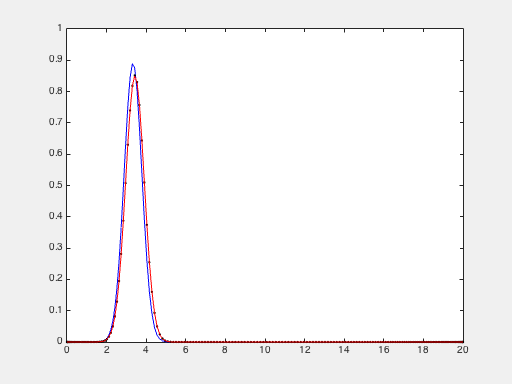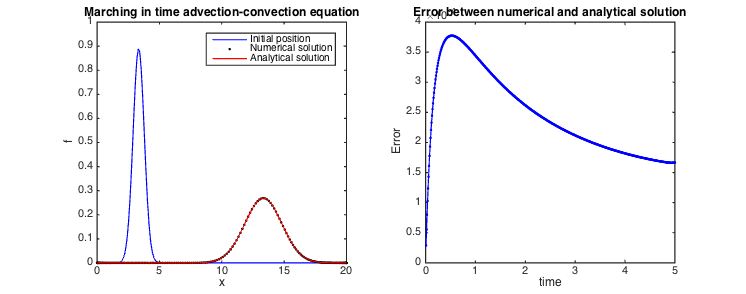# March in time of the vibrating string: f_t+Uf_x=\mu f_{xx}

Here, we studythe marching in time of the advection-diffusion equation. For solve it, we use the code build upon vibration_string

clear all; clf

% parameters
N=200; % number of gridpoints
L=20; % domain length
U=2; % wave velocity
dt=0.01; % time step
tmax=5; % final time
mu=0.2; %thermic conductivity
x0=L/6; %x-coordinate at initial time
l0=0.5; %length width
t0=0.5; %time for starting Dirac

% differentiation matrices
scale=-2/L;
[x,DM] = chebdif(N,2);
dx=DM(:,:,1)*scale;
dxx=DM(:,:,2)*scale^2;
x=(x-1)/scale;
Z=zeros(N,N); I=eye(N);


# System matrices

Here we build the matrices that give the discretization of the equations. The equations is \displaystyle f_t+Uf_x=\mu f_{xx} This is an equation with a single derivative and a double derivative, we can write this equation in the following way \displaystyle f_t=-UD_xf+\mu D_xxF

Now, we can transform this equation in a linear system with the form \displaystyle Ef_{t}=Af

% system matrices
E=I;
A=-U*dx+mu*dxx;

% boundary conditions
E([1 N],:)=0;
A([1 N],:)=I([1 N],:);


# March in time

Here we use the same method as vibrating_string.m.

% march in time matrix
M=(E-A*dt/2)\(E+A*dt/2);

% initial condition
q=(exp(-(x-x0).^2/(4*mu*t0)))/(2*sqrt(pi*mu*t0));
q0=q; %save of initial condition

% marching loop
tvec=dt:dt:tmax;
Nt=length(tvec);
e=zeros(Nt,1);

n1=1; %for 1st frame for gif
figure(1)
filename = 'advection-diffusion_marching_time.gif'; %name of gif
for ind=1:Nt
t=ind*dt;
q=M*q; % one step forward
qt=(exp(-(x-U*t-x0).^2/(4*mu*(t0+t))))/(2*sqrt(pi*mu*(t0+t))); %analytical solution
err(ind)=norm(q(:)-qt(:),2); %calculate error

% plotting
plot(x,q0,'b',x,q,'.k',x,qt,'r');
axis([0,L,0,1])
drawnow

%creat file in gif
frame = getframe(1);
im = frame2im(frame);
[A,map] = rgb2ind(im,256);
if n1 == 1;
imwrite(A,map,filename,'gif','LoopCount',Inf,'DelayTime',0.05);
n1=0;
else
imwrite(A,map,filename,'gif','WriteMode','append','DelayTime',0.05);
end
endMarching in time of advection-diffusion with numerical and analytical solution

legend('Initial position','Numerical solution','Analytical solution');
xlabel('x'); ylabel('f');

set(gcf,'paperpositionmode','auto')


# Validation

To validate the solution, we draw the error between numerical and analytical solution.

figure(2)
plot(tvec,err,'b.-');
title('Error between numerical and analytical solution');
xlabel('time'); ylabel('Error');

set(gcf,'paperpositionmode','auto')